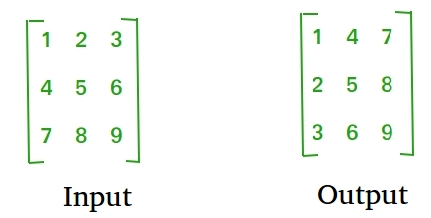Related Articles

# Java Program to Display Transpose Matrix

• Difficulty Level : Expert
• Last Updated : 07 Jan, 2021

Transpose of a matrix is obtained by changing rows to columns and columns to rows. In other words, transpose of A[][] is obtained by changing A[i][j] to A[j][i].Attention reader! Don’t stop learning now. Get hold of all the important Java Foundation and Collections concepts with the Fundamentals of Java and Java Collections Course at a student-friendly price and become industry ready. To complete your preparation from learning a language to DS Algo and many more,  please refer Complete Interview Preparation Course.

Approach:

• Create a 2-D Array.
• Insert the values in the array by running two nested loops. The outer ith loop will go till the no.of rows and the inner jth loop will run till the number of columns.
• For displaying the transpose of the matrix, run the same loop as explained in the above step but print the a[j[i] th element every time we are traversing inside the loop.

Example:

## Java

 `// Java Program to Display Transpose Matrix`` ` `import` `java.util.*;``public` `class` `GFG {``    ``public` `static` `void` `main(String args[])``    ``{``        ``// initialize the array of 3*3 order``        ``int``[][] arr = ``new` `int``[``3``][``3``];`` ` `        ``System.out.println(``"enter the elements of matrix"``);`` ` `        ``int` `k = ``1``;`` ` `        ``// get the elements from user``        ``for` `(``int` `i = ``0``; i < ``3``; i++) {``            ``for` `(``int` `j = ``0``; j < ``3``; j++) {``                ``arr[i][j] = k++;``            ``}``        ``}`` ` `        ``System.out.println(``"Matrix before Transpose "``);`` ` `        ``// display original matrix``        ``for` `(``int` `i = ``0``; i < ``3``; i++) {``            ``for` `(``int` `j = ``0``; j < ``3``; j++) {``                ``System.out.print(``" "` `+ arr[i][j]);``            ``}``            ``System.out.println();``        ``}`` ` `        ``System.out.println(``"Matrix After Transpose "``);`` ` `        ``// transpose and print matrix``        ``for` `(``int` `i = ``0``; i < ``3``; i++) {``            ``for` `(``int` `j = ``0``; j < ``3``; j++) {``                ``System.out.print(``" "` `+ arr[j][i]);``            ``}``            ``System.out.println();``        ``}``    ``}``}`
Output
```enter the elements of matrix
Matrix before Transpose
1 2 3
4 5 6
7 8 9
Matrix After Transpose
1 4 7
2 5 8
3 6 9```

My Personal Notes arrow_drop_up